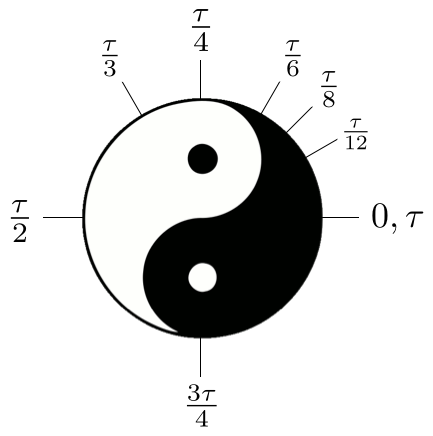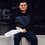# Half Tau Day is Fast Approaching!Hello all! I hope you're all gearing up for $$\frac{\tau}{2} = \pi$$ day! I am a giant proponent of $$\tau$$, and you should be too. For those of you who don't know, $$\tau = 2\pi$$. $$\tau$$ is a much more natural circle constant, as a circle is, after all, defined by its radius, not its diameter. One can find an incredibly compelling argument for $\tau$ in Michael Hartl's Tau Manifesto. I know I'll be spending Half Tau Day unwillingly participating in $\pi$ activities with my blissfully ignorant peers, who I will inevitably urge to join Brilliant and educate themselves about the marvels of $\tau$!

Have a safe and happy $\frac{\tau}{2}$ day!Note by Ryan Tamburrino
6 years, 5 months ago

This discussion board is a place to discuss our Daily Challenges and the math and science related to those challenges. Explanations are more than just a solution — they should explain the steps and thinking strategies that you used to obtain the solution. Comments should further the discussion of math and science.

When posting on Brilliant:

• Use the emojis to react to an explanation, whether you're congratulating a job well done , or just really confused .
• Ask specific questions about the challenge or the steps in somebody's explanation. Well-posed questions can add a lot to the discussion, but posting "I don't understand!" doesn't help anyone.
• Try to contribute something new to the discussion, whether it is an extension, generalization or other idea related to the challenge.

MarkdownAppears as
*italics* or _italics_ italics
**bold** or __bold__ bold
- bulleted- list
• bulleted
• list
1. numbered2. list
1. numbered
2. list
Note: you must add a full line of space before and after lists for them to show up correctly
paragraph 1paragraph 2

paragraph 1

paragraph 2

[example link](https://brilliant.org)example link
> This is a quote
This is a quote
    # I indented these lines
# 4 spaces, and now they show
# up as a code block.

print "hello world"
# I indented these lines
# 4 spaces, and now they show
# up as a code block.

print "hello world"
MathAppears as
Remember to wrap math in $$ ... $$ or $ ... $ to ensure proper formatting.
2 \times 3 $2 \times 3$
2^{34} $2^{34}$
a_{i-1} $a_{i-1}$
\frac{2}{3} $\frac{2}{3}$
\sqrt{2} $\sqrt{2}$
\sum_{i=1}^3 $\sum_{i=1}^3$
\sin \theta $\sin \theta$
\boxed{123} $\boxed{123}$

Sort by:

I feel stupid for asking this but isn't $\pi$ day on $14$ March?

- 6 years, 5 months ago

Yeah! This $\pi$ day is special though, because it will be (in the American format of month/day/year) $3/14/15$. $\tau$ will have its revenge on $6/28/31$!

- 6 years, 5 months ago

To be honest they are equally valid, as $\pi$ is better in some situations and $\tau$ is better in others. For example: circumference = $\tau$r may be easier to deal but then you end up with an area of: $\frac{\tau}{2}$$r^{2}$. You may also look at it from a trigonometry point of view... all the trigonometric functions repeat themselves every $\tau$ radians, but then when proving that $\zeta$(2) = $\frac{\pi^2}{6}$ you need $\pi$to obtain: $\frac{sin(x)}{x} = \displaystyle\prod_{n=1}^{\infty} (1-(\frac{n\pi }{x})^2)$ and the argument continues....

- 6 years, 5 months ago

I will concede a few small victories to $\pi$, including that one that you mentioned regarding the $\zeta$ function. However, if you look at the Tau Manifesto, Hartl shows that area of a circle with $\tau$ follows other quadratic forms of other formulas from physics. So, that $\frac{1}{2}$ out in front isn't quite as unnatural as it seems.

- 6 years, 5 months ago

Maybe there ought to be a friendly Tau vs pi debate

- 6 years, 5 months ago